# NCERT Solutions for Class 10 Maths Chapter 12 Areas Related to Circles Exercise 12.2

In this page we have NCERT Solutions for Class 10 Maths Chapter 12 Areas Related to Circles for Exercise 12.2 . Hope you like them and do not forget to like , social share and comment at the end of the page.
Things to Remember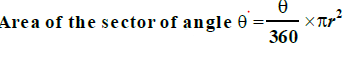Length of the arc of angle q = (q/360) × 2πr
Unless stated otherwise, use π =22/7.
Question 1
Find the area of a sector of a circle with radius 6 cm if angle of the sector is 60°.
Solution
We know that
Area of the sector making angle θ = (θ/360°)×π r
Area of the sector making angle 60° = (60°/360°)×π rcm2
= (1/6)×62π  = 6 × 22/7 cm= 132/7 cm2

Question 2
Find the area of a quadrant of a circle whose circumference is 22 cm.
Solution
We know that,
Circumference of the circle = 2πr = 22 cm
So, Radius of the circle = r = 22/2π cm = 7/2 cm
Now Quadrant of a circle means sector is making angle 90°.
Area of the sector making angle 90° = (90°/360°)×π rcm2
= (1/4)×(7/2)2π  = (49/16) × (22/7) cm= 77/8 cm2

Question 3
The length of the minute hand of a clock is 14 cm. Find the area swept by the minute hand in 5 minutes.
Solution
Here, Minute hand of clock acts as radius of the circle.
Therefore, Radius of the circle (r) = 14 cm
Angle rotated by minute hand in 1 hour = 360°
Therefore, Angle rotated by minute hand in 5 minutes = 360° × 5/60 = 30°
Area of the sector making angle 30° = (30°/360°)×π rcm2
= (1/12) × 142π  = 196/12 π cm2 = (49/3) × (22/7) cm= 154/3 cm2
Area swept by the minute hand in 5 minutes = 154/3 cm2

Question 4
A chord of a circle of radius 10 cm subtends a right angle at the centre. Find the area of the corresponding:
(i) minor segment
(ii) major sector. (Use π = 3.14)
Solution
Given Radius of the circle = 10 cm
i) Height of ΔAOB = OA = 10 cm (radius of the circle)
Base of ΔAOB = OB = 10 cm (radius of the circle)
Area of ΔAOB = 1/2 × OA × OB [As Area = (1/2) Base X Height]
= 50 cm2
inor segment is making 90°
So, Area of the sector making angle 90°
= (90°/360°) × π rcm2
= 25 × 3.14 cm= 78.5 cm2
Area of the minor segment = Area of the sector making angle 90° - Area of ΔAOB
= 78.5 cm-  50 cm= 28.5 cm2
ajor segment is making 360° - 90° = 270°
Area of the sector making angle 270°
= (270°/360°) × π rcm2
= 75 × 3.14 cm= 235.5 cm2
Therefore, Area of the major segment = 235.5 cm2
Question 5
In a circle of radius 21 cm, an arc subtends an angle of 60° at the centre. Find:
(i) the length of the arc
(ii) area of the sector formed by the arc
(iii) area of the segment formed by the corresponding chord
Solution
Given Radius of the circle = 21 cm
(i) As known from the Formula
Length of the arc = θ/360° × 2πr
= 60°/360° × 2 × 22/7 × 21
= 22 cm
(ii) Angle subtend by the arc = 60°
Area of the sector making angle 60° = (60°/360°) × π rcm2
= 441/6 π cm2
= 231 cm2

(iii) Area of equilateral ΔAOB = √(3)/4 × (OA)= √3/4 × 21= (441√3)/4 cm2
Area of the segment formed by the corresponding chord
= Area of the sector formed by the arc - Area of equilateral ΔAOB
= 231 cm- (441√3)/4 cm

Question 6
A chord of a circle of radius 15 cm subtends an angle of 60° at the centre. Find the areas of the corresponding minor and major segments of the circle. (Use π = 3.14 and √3 = 1.73)
Solution
The problem is depicted below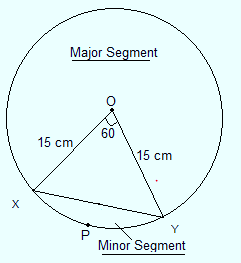Radius of the circle = 15 cm
i) ΔXOY is isosceles as two sides are equal.
Therefore, ∠X = ∠Y
Sum of all angles of triangle = 180°
∠X + ∠O + ∠Y = 180°
2 ∠X = 180° - 60°
∠X = 60°
Therefore, Triangle is equilateral as ∠X = ∠Y = ∠O = 60°
Therefore, OX = OY = XY = 15 cm
Area of equilateral ΔXOY = √(3)/4 × (OX)= √3/4 × 15
= (225√3)/4 cm= 97.3 cm2

Angle subtend at the centre by minor segment = 60°
Area of Minor sector making angle 60° = (60°/360°) × π rcm2
= (1/6) × 15π  cm2 =  225/6 π  cm2
=  (225/6) × 3.14 cm= 117.75  cm2

Area of the minor segment = Area of Minor sector - Area of equilateral ΔXOY
= 117.75 - 97.3= 20.4 cm2

Angle made by Major sector = 360° - 60° = 300°
Area of the sector making angle 300° = (300°/360°) × π rcm2
= (5/6) × 15π   =1125/6 π   cm2
= 588.75   cm2

Area of major segment = Area of Major sector + Area of equilateral ΔXOY
= 588.75+ 97.3 = 686.05 cm2

Question 7
A chord of a circle of radius 12 cm subtends an angle of 120° at the centre. Find the area of the corresponding segment of the circle. (Use π = 3.14 and √3 = 1.73)
Solution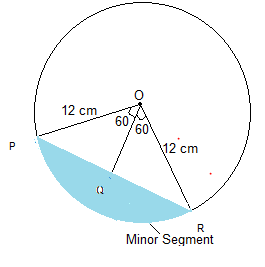Radius of the circle, r = 12 cm
Draw a perpendicular OQ to chord PR. It will bisect PR.
In ΔOPQ
∠P = 180° - (90° + 60°) = 30°
cos 30° = PQ/OP
√3/2 = PQ/12
PQ = 6√3 cm
PR = 2 × PQ = 12√3 cm
sin 30° = OQ/OP
1/2 = OQ/12
OQ = 6 cm
Area of ΔPOR = 1/2 × base × height
= 1/2 × 12√3 × 6 = 36√3 cm
= 36 × 1.73 = 62.28 cm2
Angle made by Minor sector = 120°
Area of the sector making angle 120° = (120°/360°) × π rcm2
= (1/3) × 12π
= 150.72  cm2
Therefore, Area of the corresponding Minor segment = Area of the Minor sector - Area of ΔPOR                                                                                                = 88.44 cm2

Question 8
A horse is tied to a peg at one corner of a square shaped grass field of side 15 m by means of a 5 m long rope .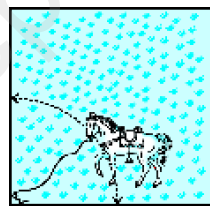Find
(i) the area of that part of the field in which the horse can graze.
(ii) the increase in the grazing area if the rope were 10 m long instead of 5 m. (Use π = 3.14)
Solution
Side of square field = 15 m
Length of rope is the radius of the circle, r = 5 m
Since, the horse is tied at one end of square field, it will graze only quarter of the field with radius 5 m.
(i) Area of circle = π r= 3.14 × 52 = 78.5 m2
Area of that part of the field in which the horse can graze = 1/4 of area of the circle = 78.5/4 = 19.625 m2
(ii) Area of circle if the length of rope is increased to 10 m = π r2 = 3.14 × 102 = 314 m2
Area of that part of the field in which the horse can graze = 1/4 of area of the circle
= 314/4 = 78.5 m2
Increase in grazing area = 78.5 m2 - 19.625 m2 = 58.875 m2

Question 9
A brooch is made with silver wire in the form of a circle with diameter 35 mm. The wire is also used in making 5 diameters which divide the circle into 10 equal sectors as shown below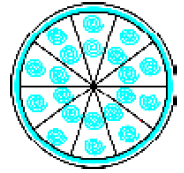Find:
(i) the total length of the silver wire required.
(ii) the area of each sector of the brooch.
Solution
Number of diameters = 5
Length of diameter = 35 mm
(i) Total length of silver wire required = Circumference of the circle + Length of 5 diameters
= 2π r + (5×35) = (2 × 22/7 × 35/2) + 175
= 110 + 175 mm = 285 mm
(ii) Number of sectors = 10
Area of each sector = Total area/Number of sectors
Total Area = π r2 = 22/7 × (35/2)= 1925/2 mm2
Therefore, Area of each sector = (1925/2) × 1/10 = 385/4 mm2

Question 10
An umbrella has 8 ribs which are equally spaced. Assuming umbrella to be a flat circle of radius 45 cm, find the area between the two consecutive ribs of the umbrella.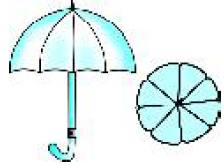Solution
Number of ribs in umbrella = 8
Radius of umbrella while flat = 45 cm
Area between the two consecutive ribs of the umbrella =
Total area/Number of ribs
Total Area = π r2 = 22/7 × (45)= 6364.29 cm2
Therefore, Area between the two consecutive ribs = 6364.29/8 cm2
= 795.5 cm2

Question 11
A car has two wipers which do not overlap. Each wiper has a blade of length 25 cm sweeping through an angle of 115°. Find the total area cleaned at each sweep of the blades.
Solution
Angle of the sector of circle made by wiper = 115°
Radius of wiper = 25 cm
Area of the sector made by wiper = (115°/360°) × π rcm2
= 23/72 × 22/7 × 25=   23/72 × 22/7 × 625 cm2
= 158125/252 cm2

Total area cleaned at each sweep of the blades = 2 ×158125/252 cm2 =  158125/126 = 1254.96 cm2

Question 12
To warn ships for underwater rocks, a lighthouse spreads a red coloured light over a sector of angle 80° to a distance of 16.5 km. Find the area of the sea over which the ships are warned.
(Use π = 3.14)
Solution
Let O bet the position of Lighthouse.
Angle made by the sector = 80°
Area of the sea over which the ships are warned = Area made by the sector.
Area of sector = (80°/360°) × π r
= 2/9 × 3.14 × (16.5)
= 189.97 km2
Question 13
A round table cover has six equal designs as shown in below figure. If the radius of the cover is 28 cm, find the cost of making the designs at the rate of ? 0.35 per cm2 . (Use √3 = 1.7)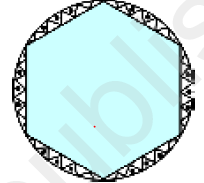Solution

Number of equal designs = 6
Radius of round table cover = 28 cm
Cost of making design = ? 0.35 per cm2
∠O = 360°/6 = 60°
ΔAOB is isosceles as two sides are equal. (Radius of the circle)
Therefore, ∠A = ∠B
Sum of all angles of triangle = 180°
∠A + ∠B + ∠O = 180°
⇒ 2 ∠A = 180° - 60°
⇒ ∠A = 120°/2
⇒ ∠A = 60°
Triangle is equilateral as ∠A = ∠B = ∠C = 60°
Area of equilateral ΔAOB = √3/4 × (OA)= √3/4 × 28= 333.2 cm2

Area of sector ACB = (60°/360°) × π rcm2
= 1/6 × 22/7 × 28 × 28 = 410.66 cm2
Area of design = Area of sector ACB - Area of equilateral ΔAOB
= 410.66 cm2 - 333.2 cm= 77.46 cm2
Area of 6 design = 6 × 77.46 cm= 464.76 cm2
Total cost of making design = 464.76 cm× ? 0.35 per cm2 = ? 162.66
Question 14
Tick the correct Solution in the following:
Area of a sector of angle p (in degrees) of a circle with radius R is
(A) p/180 × 2πR
(B) p/180 × π R2
(C) p/360 × 2πR
(D) p/720 × 2πR
Solution
Area of a sector of angle p = p/360 × π R2
= p/360 × 2/2 × π R
=  2p/720 × 2πR
Hence, Option (D) is correct.Go back to Class 10 Main Page using below links

### Practice Question

Question 1 What is $1 - \sqrt {3}$ ?
A) Non terminating repeating
B) Non terminating non repeating
C) Terminating
D) None of the above
Question 2 The volume of the largest right circular cone that can be cut out from a cube of edge 4.2 cm is?
A) 19.4 cm3
B) 12 cm3
C) 78.6 cm3
D) 58.2 cm3
Question 3 The sum of the first three terms of an AP is 33. If the product of the first and the third term exceeds the second term by 29, the AP is ?
A) 2 ,21,11
B) 1,10,19
C) -1 ,8,17
D) 2 ,11,20